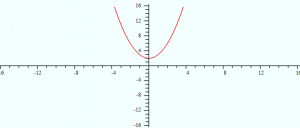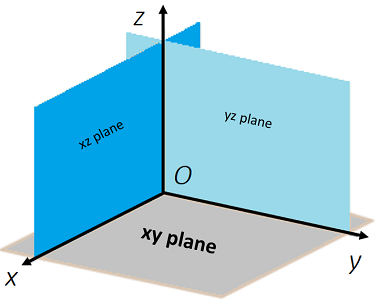# Analytic Geometry

Analytic geometry creates a connection between graphs and equations. For example, the linear function f(x) = x2 – 2 (an equation) can also be represented by a graph:Euclidean Geometry is based solely on geometric axioms without formulas or co-ordinates; Analytic geometry is the “marriage” of algebra and geometry with axes and co-ordinates .

## Calculus and Analytic Geometry

Calculus and analytic geometry have become so intertwined, it’s rare nowadays to find a course in pure “Analytic Geometry”. It’s more common to take a course in Calculus and Analytic Geometry, which blends the principles of basic analytic geometry with concepts like functions, limits, continuity, derivatives, antiderivatives, and definite integrals.

Curves:

## X Y Plane

In 3D space (also called xyz space), the xy plane contains the x-axis and y-axis:The xy plane can be described as the set of all points (x, y, z) where z = 0. In other words, any point (x, y, 0). For example, all of the following points are on the xy plane:

• (1, 5, 0)
• (-2, 19, 0)
• (π, -1, 0)
• (.5, .2, 0)

This fact gives us the equation for the xy plane: z = 0.

This is just an extension of the same idea of the x-axis (in the Cartesian plane) being the place where y = 0:(Left) The x-axis in 2D is where points are located at y = 0. In 3D, the xy plane has points where z = 0.

The xy plane, together with the yz plane and xz plane, divide space into eight octants. The O in the center of the diagram is the origin, which is a starting point for the 3D-coordinate system. The points are described by an ordered triple of real numbers (x, y, z). For example, the point (2, 3, 0) can be found at:

• x = 2,
• y = 3,
• z = 0.

As z is zero, we know this point must be somewhere on the xy plane.

## Distance Formula for Points in the XY Plane

The distance between any two points in xyz-space can be found with a generalization of the distance formula:Example question: What is the distance between the points (4, 3, 0) and (2, 9, 0)?

Step 1: Identify the coordinate components that we need to put into the formula. We know our coordinates are always ordered (x, y, z), so:

1. (4, 3, 0):
• x1 = 4
• y1 = 3
• z1 = 0.
2. (2, 9, 0):
• x2 = 2
• y2 = 9
• zz = 0.

Don’t worry about which coordinate is which (e.g. does x = 4 go into x1 or x2?). The distance formula squares these values, so you’ll get the same answer no matter which way you choose.

Step 2: Plug your values from Step 1 into the distance formula:If you aren’t good with algebra, head over to Symbolab and just replace the x, y, z values with your inputs.

## References

 Analytic Geometry and Calculus. Retrieved May 3, 2021 from: math.uci.edu/~ndonalds/math184/analytic.pdf

CITE THIS AS:
Stephanie Glen. "Analytic Geometry" From StatisticsHowTo.com: Elementary Statistics for the rest of us! https://www.statisticshowto.com/calculus-problem-solving/analytic-geometry/